# The equation III-th degree

## How do you solve an equation of the third degree with real roots

An equation of the third degree has the form:

,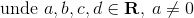To solve this equation we use the following substitution: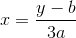Then we have: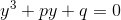, where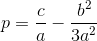,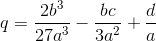We note: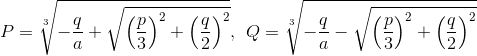The roots of this equation are: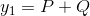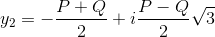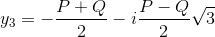### The Discriminant of the equation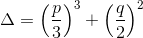1.  If Δ < 0: The equation has 3 distinct real roots (solutions).

2.  If Δ = 0: The equation has three real roots, of which at least two are the same.

3.  If Δ > 0: The equation has only one real root and two complex roots, combined.

_

Keywords: equations, equation, algebra, maths, 3rd grade, grade 3, superior algebra

### Forum

Here you can discuss about mathematic, about algebra, geometry, trigonometry.

It is not mandatory to be logged in on this forum but it is nice to have an account. You can ask about mathematics just with your name and your email.

This maths forum is one of the easiest forums to use it.

>> Go to Math Forum